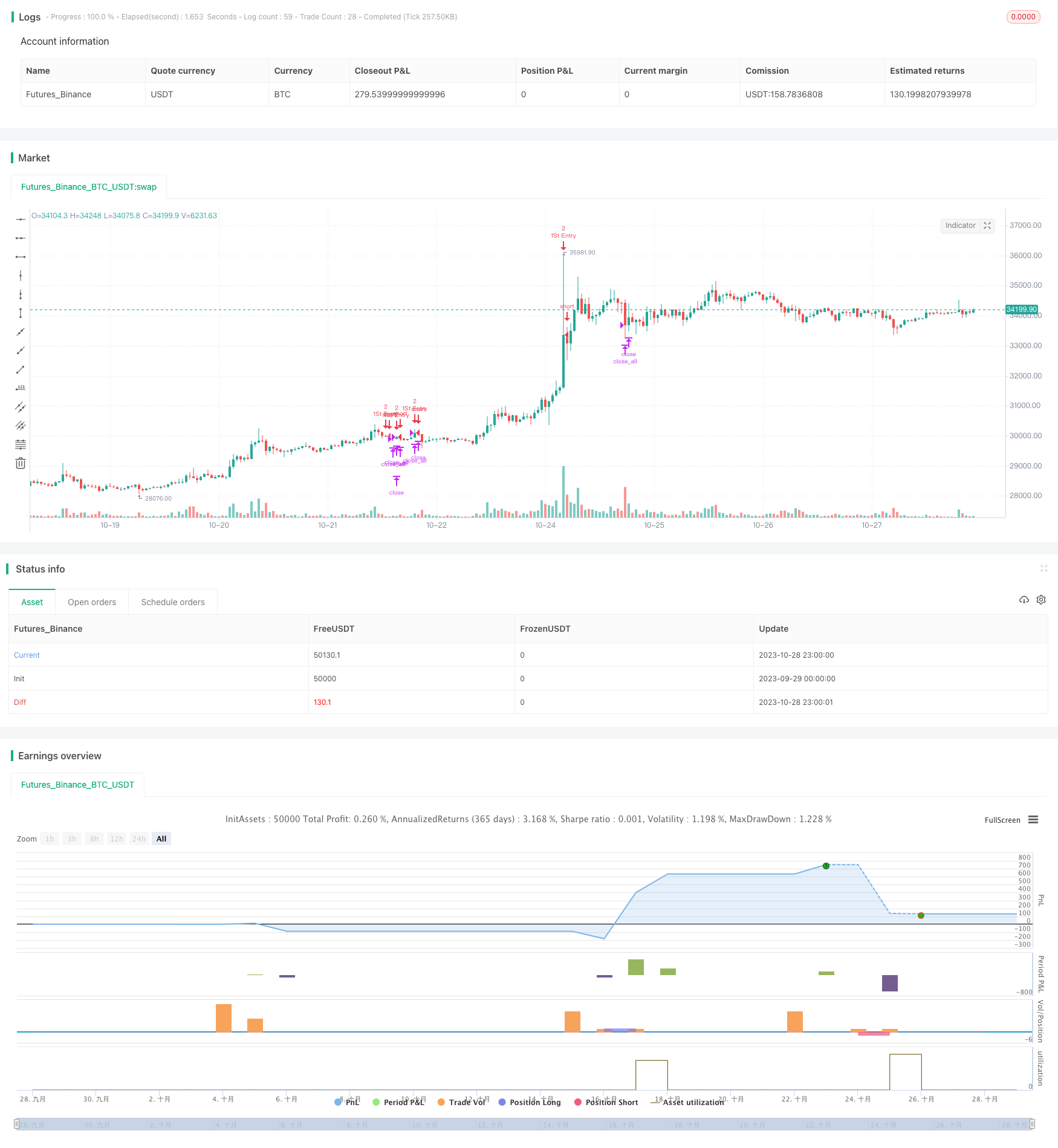# RSI Long Short Balance Trading Strategy

Author: ChaoZhang, Date: 2023-10-30 15:49:35
Tags:### Overview

This strategy uses a combination of RSI indicators across different timeframes to determine if the current market is overbought or oversold, and combines the relationship between price and moving average to generate buy and sell signals. The goal is to buy on dips and sell on rallies to profit during consolidation.

### Strategy Logic

1. Calculate the RSI values of the 5-minute, 15-minute, and 1-hour timeframes. When the 5-minute, 15-minute and 1-hour RSI are all below 25 at the same time, it is judged as an oversold condition and generates a buy signal. When the 5-minute, 15-minute and 1-hour RSI are all above 75 at the same time, it is judged as an overbought condition and generates a sell signal.

2. Breaking the 21-day moving average also acts as a trading signal. If the price is below the moving average, a buy signal is generated. If the price is above the moving average, a sell signal is generated.

3. Based on the current position, the initial trade size and pyramiding rules are set: 2 contracts for the first entry, and then adding 1 contract each time until the position reaches 2 contracts.

4. The stop loss is triggered when the loss reaches 3%. Take profit when the profit reaches 1%.

1. Using RSI indicators across multiple timeframes to determine overbought and oversold conditions improves signal reliability.

3. Setting position sizing control and profit/loss ratio for stop loss and take profit manages risks.

4. Scaling in with fixed quantity expands profit potential.

### Risks

1. RSI divergence risk. Price may continue to trend for a period after RSI reaches overbought or oversold threshold before reversing. Blindly following RSI signal can lead to losses.

2. Moving average trading signal may be misleading. Moving average fails to track price change timely during huge price swings.

3. Incorrect position sizing and profit/loss ratio settings lead to improper risk control.

4. Pyramiding conditions need to be set reasonably to avoid magnifying losses.

### Optimization Directions

1. Adjust RSI parameters and test different period combinations to find more reliable overbought/oversold signals.

2. Test different moving averages as auxiliary trading signals, or other technical indicators.

3. Optimize position sizing and stop loss/take profit rules to build more scientific risk control mechanisms.

4. Optimize pyramiding conditions to prevent magnifying losses. Consider exponential scaling instead of fixed quantity scaling.

### Summary

This strategy uses RSI across multiple timeframes to determine trend potential and achieve higher win rate. Additional signals are generated with moving averages to expand trading opportunities. Risk is managed through position sizing, stop loss/take profit, and fixed quantity pyramiding. Overall, this strategy combines trend and mean reversion indicators, incorporates both trend following and bottom-picking logic, and is effective during consolidation. Further testing and optimization is needed to build more robust risk control for more consistent performance.

```/*backtest
start: 2023-09-29 00:00:00
end: 2023-10-29 00:00:00
period: 1h
basePeriod: 15m
exchanges: [{"eid":"Futures_Binance","currency":"BTC_USDT"}]
*/

//@version=3
strategy("5M_RSI_Strategy", overlay=true, pyramiding = 1)
len =14
up = rma(max(change(close), 0), len)
down = rma(-min(change(close), 0), len)
rsi = down == 0 ? 100 : up == 0 ? 0 : 100 - (100 / (1 + up / down))
RSI_1h = request.security(syminfo.tickerid, "60", rsi)
RSI_3h = request.security(syminfo.tickerid, "180", rsi)
RSI_15m = request.security(syminfo.tickerid, "15", rsi)
RSI_5m = request.security(syminfo.tickerid, "5", rsi)
RSI_1m = request.security(syminfo.tickerid, "1", rsi)
ema21_5 = ema(request.security(syminfo.tickerid, "5", close), 21)
ema21_15 = ema(request.security(syminfo.tickerid, "15", close), 21)
//(RSI_3h<=25) and  (RSI_1h<=25) and (RSI_15m<=25) and
Positive = ((RSI_5m<=25) and (RSI_15m<=25) and (RSI_1h<=25))?true:false
//plotshape(Positive, style=shape.triangleup,location=location.belowbar, color=green,size =size.tiny)
Negative = (( RSI_5m>=75) and ( RSI_15m>=75) and ( RSI_1h>=75))?true:false
//plotshape(Negative, style=shape.triangledown,location=location.abovebar, color=red,size=size.tiny)          Positive and   Negative and

//lastordersize =1
// and ((ema21_15-low)/ema21_15) > 0.077
if (abs(strategy.position_size) >= Initial_Trade_Size and (abs(close - strategy.position_avg_price)/abs(strategy.position_avg_price)>0.03))
if(strategy.position_avg_price > close and strategy.position_size > 0)
strategy.entry("Add", strategy.long , qty = lastordersize , when = true)
if(strategy.position_avg_price < close and strategy.position_size < 0)
strategy.entry("Add", strategy.short, qty = lastordersize , when = true)
if (strategy.position_size == 0)
if (Positive or ((ema21_5-low)/ema21_5) > 0.07)
strategy.entry("1St Entry", strategy.long , qty = lastordersize , when = true)
// and ((high-ema21_15)/ema21_15) > 0.077
if (Negative or ((high-ema21_5)/ema21_5) > 0.07)
strategy.entry("1St Entry", strategy.short, qty = lastordersize , when = true)
//lastordersize := lastordersize * 2
//or (strategy.openprofit / abs(strategy.position_size * close))>=0.01
if(cross(ema21_5, high) or cross(ema21_5, low))
strategy.close_all()
```

More